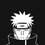# RMO #1990

After seeing that my friends on Brilliant are eagerly preparing for RMO, I wish to help them and therefore I am posting the RMO$(1990)$ question paper, I also want to know about the correct way of solving these problems (my second purpose for posting these questions). Please post solution also.

$1)$Two boxes contain between them $65$ balls of several different sizes. Each ball is white, black, red or yellow. If you take any five balls of the same colour, at least $2$ of them will always be of the same size (radius). Prove that there are at least three balls which lie in the same box have the same colour and have the same size (radius).

$2)$For all positive real numbers $a, b, c$ prove that $\frac{a}{b+c}+\frac{b}{a+c}+\frac{c}{a+b} \geq \frac{3}{2}$.

$3)$A square sheet of paper $ABCD$ is so folded that $B$ falls on the mid-point of $M$ of $CD$. Prove that the crease will divide $BC$ in the ratio $5:3$.

$4)$Find the remainder when $2^{1990}$ is divided by $1990$.

$5)$$P$ is any point inside a $\triangle ABC$. The perimeter of the triangle $AB +BC +CA=2s$. Prove that $s < AP + BP + CP < 2s.$

$6)$$N$ is a $50$-digit number (in a decimal scale). All digits except the $26^{th}$ digit (from the left) are 1.If $N$ is divisible by $13$, find the $26^{th}$ digit.

$7)$A census-man on duty visited a house in which the lady inmates declined to reveal their individual ages, but said “We do not mind giving you the sum of the ages of any two ladies you may choose”. There upon the census man said, “In that case, please give me the sum of the ages of every possible pair of you”. They gave the sums as follows: $30, 33, 41, 58, 66, 69$. The census-man took these figures and happily went away. How did he calculate the individual ages of the ladies from these figures?

$8)$If the circumcenter and centroid of a triangle coincide, prove that the triangle must be equilateral.Note by Akshat Sharda
5 years, 9 months ago

This discussion board is a place to discuss our Daily Challenges and the math and science related to those challenges. Explanations are more than just a solution — they should explain the steps and thinking strategies that you used to obtain the solution. Comments should further the discussion of math and science.

When posting on Brilliant:

• Use the emojis to react to an explanation, whether you're congratulating a job well done , or just really confused .
• Ask specific questions about the challenge or the steps in somebody's explanation. Well-posed questions can add a lot to the discussion, but posting "I don't understand!" doesn't help anyone.
• Try to contribute something new to the discussion, whether it is an extension, generalization or other idea related to the challenge.

MarkdownAppears as
*italics* or _italics_ italics
**bold** or __bold__ bold
- bulleted- list
• bulleted
• list
1. numbered2. list
1. numbered
2. list
Note: you must add a full line of space before and after lists for them to show up correctly
paragraph 1paragraph 2

paragraph 1

paragraph 2

[example link](https://brilliant.org)example link
> This is a quote
This is a quote
    # I indented these lines
# 4 spaces, and now they show
# up as a code block.

print "hello world"
# I indented these lines
# 4 spaces, and now they show
# up as a code block.

print "hello world"
MathAppears as
Remember to wrap math in $$ ... $$ or $ ... $ to ensure proper formatting.
2 \times 3 $2 \times 3$
2^{34} $2^{34}$
a_{i-1} $a_{i-1}$
\frac{2}{3} $\frac{2}{3}$
\sqrt{2} $\sqrt{2}$
\sum_{i=1}^3 $\sum_{i=1}^3$
\sin \theta $\sin \theta$
\boxed{123} $\boxed{123}$

Sort by:

It is easy to use the collory of C-S for Q2. however here is the solution with AM-HM: $\dfrac{a}{b+c}+\dfrac{b}{a+c}+\dfrac{c}{a+b} \geq \dfrac{3}{2}$, $\dfrac{a}{b+c}+1+\dfrac{b}{a+c}+1+\dfrac{c}{a+b}+1-3$ $(a+b+c)(\dfrac{1}{b+c}+\dfrac{1}{a+c}+\dfrac{1}{a+b}) -3$ $\dfrac{1}{2}[(a+b)+(b+c)+(a+c)][\dfrac{1}{b+c}+\dfrac{1}{a+c}+\dfrac{1}{a+b}]-3$ Apply AM-HM to find that $\frac{1}{2}[(a+b)+(b+c)+(a+c)][\dfrac{1}{b+c}+\dfrac{1}{a+c}+\dfrac{1}{a+b}]-3 \geq \dfrac{1}{2}[3^2]-3=\dfrac{9}{2}-3=\dfrac{3}{2}$

- 5 years, 9 months ago

Solution of Q.5

We know that in a triangle (sum of two sides) $>$ (third side)

In $\triangle APB, AP + PB>AB$

similarly, In $\triangle APC, AP + PC>AC$ and In $\triangle BPC, BP + PC>BC$

Adding the above inequalitiees we get $2(AP+BP+CP)>(AB+BC+AC) \Rightarrow (AP+BP+CP)>\frac{(AB+BC+AC)}{2} \Rightarrow (AP+BP+CP)>s$

Now, it is easy to see that

$\angle APB>\angle BAP , \angle APB>\angle ABP$

$\Rightarrow AB>AP$ and $AB>BP$

Similar reasoning gives us

$AC>AP , AC>PC$ and $BC>BP, BC>PC$

Adding we get $2(AP+BP+CP)<2(AB+BC+AC) \Rightarrow (AP+BP+CP)<2s$

$\therefore s<(AP+BP+CP)<2s$, as desired

- 3 years, 10 months ago

RMO then contained 8 questions!

- 5 years, 9 months ago

This is probably one of the easiest RMO.

- 5 years, 9 months ago

@Akshat Sharda Thanks for posting! And of course keep posting! :)

- 5 years, 9 months ago

2) Immediate using Titu's Lemma

4) Remainder is $1024$

- 5 years, 9 months ago

Q7 Ages are 47 ,22 ,19 and 11

- 4 years, 10 months ago

Q6 it is 3

- 4 years, 10 months ago

First was easy, did contradiction. You can use pigeon hole too. 2nd easy Titu Lema... 3rd easy Pythagoras 4th Fermat's little theorem and a clever observation that $2¹⁹⁹⁰-2¹⁰$ is divisible by 10 5th medium problem. Had to proof another result beforehand. 6th Really easy! Divisibility rule of 13 or you could see a nice pattern in residues $modulo 13$ for 10^n. They repeat after every 6qth power of 10. 7th Medium. One just needs to pay attention and do not assign any equation to any sum. Take assumption that she is oldest, then she is 2nd oldest bla bla... 8th problem Tooooo easy! Took hardly 5 minutes. Use basic definition of centroid, circumcenter. Properties of median, Pythagoras (at least I used) Properties of isoceles triangle. Congruency can help too I think. That's it. Give it a try.

- 10 months ago